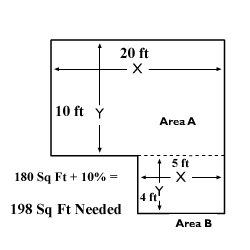top of page

# How To Measure Flooring

Make an investment that will pay off for years to come. Start by measuring your space.Measure the amount of flooring you need, with extra included, using our useful tricks.

Tools/Material

• Tape measure

• Paper

• Pen

• Calculator

Step by Step Instructions

Step 1

Measure the width and depth of your rooms.

• List the rooms you will be adding new flooring

• Measure the farthest wall distance; round up to the nearest foot

• Measure the wall distance of the other two walls; round up to the nearest foot

• Multiply the two numbers; this will be the rough estimate

• Example: Width 20 ft; Depth 10 ft; 20 x 10 = 200 sq ftStep 2 Subtract the floor space of un-movable objects -- i.e. Kitchen Islands

• Find the square footage of the object (width x depth)

• Take the total area and subtract the items square footage

• Example: Total 200 sq ft - Island is 20 sq ft = 180 sq ft

Step 3

Add the total areas of the rooms you measured

• Example: Room 1 180 sq ft + Room 2 110 sq ft + Room 3 110 sq ft = 400 sq ft

Step 4

Factor in 10 percent waste

• This will ensure you have enough especially because cuts are specific to space

• Example: 400 sq ft x .10 = 40 + 400 = 440 sq ft needed

bottom of page